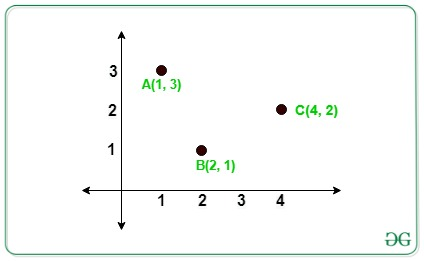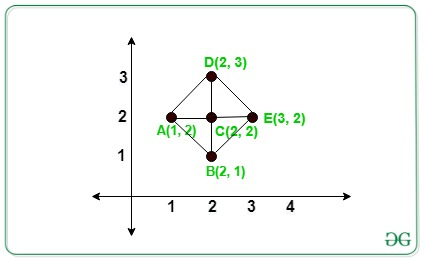# Count of Right-Angled Triangle formed from given N points whose baseA Computer Science portal for geeks. It contains well written, well thought and well explained computer science and programming articles, quizzes and practice/competitive programming/company interview Questions.

Given an array arr[] of N distinct integers points on the 2D **Plane. The task is to count the number of Right-Angled Triangle from N** points such that base or perpendicular is parallel to the X or Y axis.

Examples:

Input:_ arr[][] = {{4, 2}, {2, 1}, {1, 3}}_

Output:_ 0_

Explanation:In the above image there is no right angled triangle formed.

Input:_ arr[][] = {{1, 2}, {2, 1}, {2, 2}, {2, 3}, {3, 2}}_

Output:_ 4_

Explanation:In the above image there are 4 right angled triangle formed by triangles _**_ACB, ACD, DCE, BCE**.

Approach: The idea is to store the count of each co-ordinates having same X and Y coordinates respectively. Now traverse each given points and the count of a right-angled triangle formed by each coordinate (X, Y) is given by:

Count of right-angled triangles = (frequencies of X coordinates – 1)_ * (frequencies of **_Y**_ coordinates – 1)_

Below are the steps:

1. Create two maps to store the count of points, one for having the same X-coordinate and another for having the same Y-coordinate.
2. For each value in the map of x-coordinate and in the map of y-coordinate choose that pair of points as pivot elements and find the frequency of that pivot element.
3. For each pivot element(say pivot) in the above step, the count of right-angled is given by:

(m1[pivot].second-1)*(m2[pivot].second-1)

1. Similarly, calculate the total possible **[right-angled triangle](https://www.geeksforgeeks.org/check-whether-right-angled-triangle-valid-not-large-sides/) for other **N points given.
2. Finally, sum all the possible triangle obtained that is the final answer.

Below is the implementation of the above approach:

• C++

`// C++ program for the above approach`

`#include <bits/stdc++.h>`

`**using**` `**namespace**` `std;`

`// Function to find the number of right`

`// angled triangle that are formed from`

`// given N points whose perpendicular or`

`// base is parallel to X or Y axis`

`**int**` `RightAngled(``**int**` `a[],` `**int**` `n)`

`{`

`// To store the number of points`

`// has same x or y coordinates`

`unordered_map<``**int**``,` `**int**``> xpoints;`

`unordered_map<``**int**``,` `**int**``> ypoints;`

`**for**` `(``**int**` `i = 0; i < n; i++) {`

`xpoints[a[i]]++;`

`ypoints[a[i]]++;`

`}`

`// Store the total count of triangle`

`**int**` `count = 0;`

`// Iterate to check for total number`

`// of possible triangle`

`**for**` `(``**int**` `i = 0; i < n; i++) {`

`**if**` `(xpoints[a[i]] >= 1`

`&& ypoints[a[i]] >= 1) {`

`// Add the count of triangles`

`// formed`

`count += (xpoints[a[i]] - 1)`

`* (ypoints[a[i]] - 1);`

`}`

`}`

`// Total possible triangle`

`**return**` `count;`

`}`

`// Driver Code`

`**int**` `main()`

`{`

`**int**` `N = 5;`

`// Given N points`

`**int**` `arr[] = { { 1, 2 }, { 2, 1 },`

`{ 2, 2 }, { 2, 3 },`

`{ 3, 2 } };`

`// Function Call`

`cout << RightAngled(arr, N);`

`**return**` `0;`

`}`

Output:

``4``

Time Complexity:_ O(N)_

Auxiliary Space:_ O(1)_

Attention reader! Don’t stop learning now. Get hold of all the important DSA concepts with the DSA Self Paced Course at a student-friendly price and become industry ready.

## Queries to count characters having odd frequency in a range [L, R]

A Computer Science portal for geeks. It contains well written, well thought and well explained computer science and programming articles, quizzes and practice/competitive programming/company interview Questions.

## Find all missing numbers from a given sorted array

A Computer Science portal for geeks. It contains well written, well thought and well explained computer science and programming articles, quizzes and practice/competitive programming/company interview Questions.

## Minimize swaps required to maximize the count of elements

A Computer Science portal for geeks. It contains well written, well thought and well explained computer science and programming articles, quizzes and practice/competitive programming/company interview Questions.

## Count of right shifts for each array element to be in its sorted position

A Computer Science portal for geeks. It contains well written, well thought and well explained computer science and programming articles, quizzes and practice/competitive programming/company interview Questions.

## Count of Array elements greater than or equal to twice the Median

A Computer Science portal for geeks. It contains well written, well thought and well explained computer science and programming articles, quizzes and practice/competitive programming/company interview Questions.